# Colleges with the lowest SAT scores in Montana

Top 9 colleges in Montana with the lowest SAT scores
Looking for the colleges with the lowest SAT scores in Montana? Well you're in luck! We've compiled a national college database and have created a list of the top 9 universities with the lowest SAT scores in Montana below. If you are not a good test taker or worried about your test scores, this list is for you. These are the schools whose applicants had the lowest average SAT scores in Montana, which means that you can get into these colleges with a lower SAT score. We also include each college's ACT scores and acceptance rate so that you can see where you would have the easiest time getting in. Read on to find out more.

## Montana State University - Northern SAT scores

The average SAT score for Montana State University - Northern is 1050.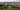The average SAT score of 1050 breaks down into:

• SAT math: 510

The average ACT score for Montana State University - Northern is 20 and their acceptance rate is 64%.

## University of Montana Western SAT scores

The average SAT score for University of Montana Western is 1060.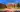The average SAT score of 1060 breaks down into:

• SAT math: 520

The average ACT score for University of Montana Western is 19 and their acceptance rate is 70%.

## Montana State University Billings SAT scores

The average SAT score for Montana State University Billings is 1070.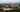The average SAT score of 1070 breaks down into:

• SAT math: 530

The average ACT score for Montana State University Billings is 20 and their acceptance rate is 100%.

## Rocky Mountain College SAT scores

The average SAT score for Rocky Mountain College is 1079.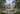The average SAT score of 1079 breaks down into:

• SAT math: 540

The average ACT score for Rocky Mountain College is 23 and their acceptance rate is 58.4%.

## University of Montana SAT scores

The average SAT score for University of Montana is 1161.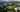The average SAT score of 1161 breaks down into:

• SAT math: 570

The average ACT score for University of Montana is 24 and their acceptance rate is 92.6%.

## Carroll College SAT scores

The average SAT score for Carroll College is 1190.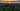The average SAT score of 1190 breaks down into:

• SAT math: 580

The average ACT score for Carroll College is 25 and their acceptance rate is 71.5%.

## Montana State University SAT scores

The average SAT score for Montana State University is 1200.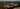The average SAT score of 1200 breaks down into:

• SAT math: 590

The average ACT score for Montana State University is 25 and their acceptance rate is 82.9%.

## Montana University System SAT scores

The average SAT score for Montana University System is 1200.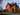The average SAT score of 1200 breaks down into:

• SAT math: 590

The average ACT score for Montana University System is 25 and their acceptance rate is 82.9%.

## Montana Tech of the University of Montana SAT scores

The average SAT score for Montana Tech of the University of Montana is 1210.The average SAT score of 1210 breaks down into:

• SAT math: 620